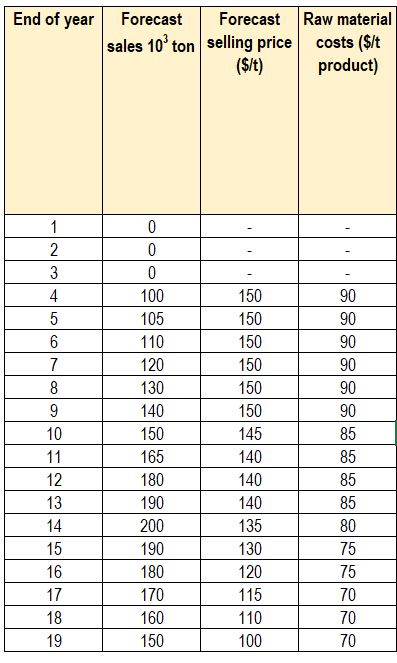# How to do Projects Economic Evaluation – Example on How to Calculate DCFRR

In this post, I want to share how to do projects economic evaluation by using example. In this example, we will learn relationship between investment, sales, raw material cost, operating cost, to get the following parameters that usually used in projects economic evaluation:

• Net cash flow
• Cumulative cash flow
• Discounted cash flow

### Example on How to do Projects Economic Evaluation

It is proposed to build a plant to produce a new product. The estimated investment required is 12.5 million dollars and the timing of the investment will be:

• Year 1    1.0 million (design costs)
• Year 2    5.0 million (construction costs)
• Year 3    5.0 million (construction costs)
• Year 4    1.5 million (working capital)

The plant will start up in year 4.

The forecast sales price, sales volume, and raw material costs are shown in table below.The fixed operating costs are estimated to be:

• $400,000 per year from year 1 to year 8 •$500,000 per year from year 9 to year 13
• $550,000 per year from year 14 to year 19 The variable operating costs are estimated to be: •$10 per ton product from year 1 to year 14
• $13 per ton product from year 15 to year 19 Calculate: 1. The net cash flow in each year 2. The future worth of the project (NFW) 3. The present worth (NPW) at a discount rate of 15% 4. The discounted cash-flow rate of return (DCFRR) 5. The pay-back time No account needs to be taken of tax in this example or the scrap value of the equipment and value of the site at the end of the project life. For the discounting calculation, cash flows can be assumed to occur at the end of the year in which they occur. ### Solution The cash-flow calculations are summarized in table below.Calculation results Sample calculations to illustrate the method used are given below. For year 4 • Investment (negative cash flow) =$ 1.5 × 106
• Sales income = 100 × 1000 × 150 = $15 × 106 • Raw material cost = 100 × 1000 × 90 =$ 9 × 106
• Fixed operating costs = $0.4 × 106 • Variable operating costs = 100 × 1000 × 10 =$ 1 × 106
• Net cash flow = sales income – costs – investment = 15 – 9 – 0.4 – 1 = $3.1 × 106 • Discounted cash flow (at 15%) = 3.1 / (1 + 0.15)4 =$ 1.77 × 106

For year 8

• Investment (negative cash flow) = 0
• Sales income = 130 × 1000 × 150 = $19.5 × 106 • Raw material cost = 130 × 1000 × 90 =$ 11.7 × 106
• Fixed operating costs = $0.4 × 106 • Variable operating costs = 130 × 1000 × 10 =$ 1.3 × 106
• Net cash flow = sales income – costs – investment = 19.5 – 11.7 – 0.4 – 1.3 = $6.1 × 106 • Discounted cash flow (at 15%) = 6.1 / (1 + 0.15)8 =$ 1.99 × 106

DCFRR or Internal Rate of Return or Interest Rate of Return is found by trial-and-error calculation. It is found when Net Present Worth is zero.  The present worth has been calculated at discount rates of 25%, 35% and 37%. By using spreadsheet, we can use goal-seek. In this example, I used goal see with the following setting.